# Investigate the Effect of Damping Parameters of the Hydrodynamic Bearings Using the Optimization Method of Design of Experiments

Investigate the Effect of Damping Parameters of the Hydrodynamic Bearings Using the Optimization Method of Design of Experiments

Naouri AbdallahRefassi Kaddour Youness Mimmoun Brihmat Mostefa

LMSS Laboratory, Department of Mechanical Engineering, University of Djillali Liabes, Sidi bel - abbes 22000, Algeria

Department of Electrical Engineering, University of Djillali Liabes, Sidi bel- abbes 22000, Algeria

Corresponding Author Email:
abdallah.naouri@univ-sba.dz
Page:
103-112
|
DOI:
https://doi.org/10.18280/mmep.070113
13 October 2019
|
Accepted:
24 February 2020
|
Published:
31 March 2020
| Citation

OPEN ACCESS

Abstract:

The dynamic behavior of fluid film bearings is one of the principal factors, which affect the performance of the rotating machines. In this research, a rigid rotor supported by two identical hydrodynamic bearings is considered. The main objective of this work is to predict the influence of the damping film of the hydrodynamic bearings on the stability of the rotating machines. Analysis of variance requested to identify the frequency of a system by the effect of damping coefficients that affects the unbalance of the system investigated by employing two software is presented, Matlab to calculate damping and the frequency of the coefficients, the design of Experiments (DOE) for calculates statistical effect of these parameters on the frequency of the system. Thus, in the DOE two levels were use in fifteen factors resulting in forty-eight combinations according to the Plakett-Burman design. The effect and Pareto graphs have studied to identify which damping coefficients and diameters are responsible for producing greatly effects on frequency and stability. The results showed that the damping coefficient Czy and D10, D17 produce major effects, on the stability of the system.

Keywords:

hydrodynamic bearing, design of experiments, stability, damping coefficient, Plakett-Burman design, rotating machines, dynamic coefficients

1. Introduction

The dynamic control of behaviors requires a prediction of the characteristics of damping of the rotors, in particular damping due to the rotating parts equipped with the hydrodynamic bearing, which can generate an instability. This prediction is fundamental in the design of the rotating machines in order to provide a precise idea of the “security” sands in terms number of critical speeds. The experiment showed that under certain operating conditions, the hydrodynamic bearings have an unstable behavior. Newkirk  identified a natural vibration with the trees supported by fluid stages. Two effects are to be specified, the first is a movement of precession of the tree at a speed close to half the number of revolutions, the second is a whipping characterized by an increase in the amplitudes at a fixed speed, near to half of the own pulsation of the rotor. This speed does not evolve/move any more with that of the tree, and it is advisable not to exceed it, to avoid the destruction of the machine. In added study, Cha  studied the dynamic behavior of a cylindrical plane bearing by employing the two numerical approaches linear and nonlinear, from where it compared the orbits of the movement of the tree obtained by the linear analysis with that nonlinear in the presence of the deformation with the stage. The knowledge of the dynamic coefficients makes it possible to the specialists in vibration to model the bearing for the research of instabilities of process. The robust design method provides a systematic and efficient approach to find the near optimum combination of design parameters that optimize the performance, the techniques of design (DOE) can provide much data on the impact of a response because of one or several factors. DOE ensures the improvement and the identification of the points of reduction, which have important effects of the factors and their response on stability and avoid the serious effects of the vibrations induced by the system., which makes it possible to have access to information and to analyze invaluable on the results .

Matlab is a powerful system which intégrâtes mathematicals fonctions for finite élément analyses and it is widely used in the research and development of the rotary machines. It has many graphic functions rotating éléments which can be employed to model the axis and a powerful system operators applying to matrices for a rotating élément, the gyroscopic effect can be taken into account. Also, the effects of rotatory inertia, the axial load and the internal damping can be included. The geometry element is can be specified by stiffness, damping or mass matrix,of rotor-bearing system are included in this paper.

The study of rotating systems resides in extent of their stability, while holding account of the possibility of manufacturing errors as the machines are subject to a balance control before setting into service, which makes the balancing of rotating systems during operation necessary to reduce vibrations . The non-linear forces are achieved in the hydrodynamic bearing studied the limit stability for a short-hydrodynamic bearing in Newton liquid [5, 6]. Some designers have studied the impact of the flexibility of the turbo machinery by using experimental data and procedures allowing to increase the effectiveness and the characteristics [7, 8]. In order to study the dynamic behavior of the rotary systems, the use of finite elements was applied to increase models adapted in the field of the dynamics of the rotors and of the hydrodynamic bearings allowed to exploit these models in order to design rotating machines employed with parameters well defined . Kaidi and Kabouche  investigated the prediction of the defects and the phenomena to which the hydrodynamic bearing is its vibratory behavior through a numerical model by using the method based on the linear model to determine the coefficients of stiffness and damping and the unbalanced response by solving the shaft’s equation of motion. The result obtained noted that the eccentricity of unbalance has an important influence on the response and that the variation of the geometrical parameters of the bearing increase the vibration amplitudes. Chouksey and Coworkers  have studied the influences of the damping of the inner rotor material and the forces of the fluid film (generated by the hydrodynamic action in the bearings) on the modal behavior of a flexible rotor-shaft system.

A study was carried on the use of the Plakett-Burman statistical method in the design of experiments in order to identify the influence effect of stiffness coefficients on the dynamics of rotating machines as well as on the diameters responsible for the production of these high frequencies . The interaction between the factor and the other can increase or decrease the main effects as confirmed by interaction graphs and surface graphs. the result show That the inclusion of the stiffness coefficients on the dynamic analysis of rotating machines supported on hydrodynamic bearings play an important rôle on the determination of the unbalance response of rotors.

Fegade and Patel  study the influence of the frequency by variation of the diameters using the methodology of design (DOE) according to the Plakett-Burman design and parametric design ANSYS,the results show that the diameters D10 and D12 have a major effect on the frequency of excitation of fluid film carrying the frequency of selected maximum excitation.

Current work presents the continuation of research who has the objective studied the influence of the parameters dynamic behavior of the bearing on its vibratory described in previous researches [12, 13]. For that, two software are introduced for the resolution of the problem and determined particularly the damping coefficients from a model to detect the damping and the diameters, which are responsible for the principal effects on the frequency. Thus, investigates the influence on the Vibration in rotating system. The harmonic analysis is to identify the frequency of the rotor through the variation of the diameters by the design of the optimization (DOE).

In the DOE, two levels are used with a total of eleven diameters and four damping factors as parameters, which made forty-eight tests according to the Plakett-Burman plan for responses. Study Pareto chart of the standardized effects and normal plot of the standardized effects and main effects plot of frequency have to identify the damping and the diameters that are responsible for producing major effects on frequency. It has been seen that the damping coefficient Czy and the diameters D10 and D17, are responsible for producing major effects on the frequency, have a significant impact on the dynamic behavior of rotating machinery.

2. Dynamic Coefficients of Fluid Bearings

The good knowledge of the dynamic characteristics of the bearing (four stiffness coefficients and four damping coefficients) make it possible to model them for the research of instability of operation and the precise analysis of system. The bearings are used for supported horizontal and verticals vibrations due to unbalance and the action of the load, the shaft center moves about an equilibrium position is described by the eccentricity, e, and the angle of eccentricity, Ф. However, the orbit of the movement of axis is small compared with the bearing clearance.

The fluid film forces are functions of displacements and speeds at the center of the journal. The dynamic displacements of journal are less than the bearing clearance, which subjected to the dynamic response of the bearing and small amplitude motion; the dynamic coefficients (stiffness and damping coefficients) are obtained. In Figure 1 a bearing is exposed to a small external force (small amplitude motion), the effect to change the load on the position of the shaft provide more displacement of Δx and Δy from this position where the resultant reaction load has components of ωx and ωy .Thus, performing a first order Taylor expansion of these components gives [14, 15]:

## 1.png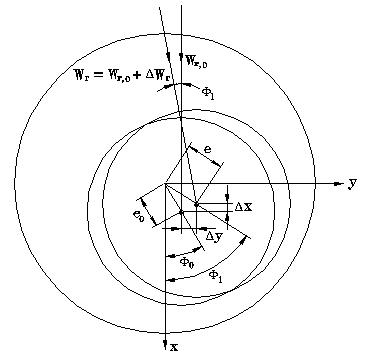Figure 1. Parameters adapted by the effect of change of the load

$\omega_{x}=\left(\omega_{x}\right)_{0}+\left(\frac{\partial \omega_{x}}{\eth x}\right)_{0} \Delta x+\left(\frac{\partial \omega_{x}}{\eth y}\right)_{0} \Delta y+\left(\frac{\partial \omega_{x}}{\partial x^{.}}\right)_{0} \Delta x^{.}+\left(\frac{\partial \omega_{x}}{\partial y^{.}}\right)_{0} \Delta y^{.}$   (1)

$\omega_{y}=\left(\omega_{y}\right)_{0}+\left(\frac{\partial \omega_{y}}{\eth x}\right)_{0} \Delta x+\left(\frac{\partial \omega_{y}}{\eth y}\right)_{0} \Delta y+\left(\frac{\partial \omega_{y}}{\partial x^{.}}\right)_{0} \Delta x^{.}+\left(\frac{\partial \omega_{y}}{\partial y^{.}}\right)_{0} \Delta y^{.}$     (2)

Due to the direction chosen for the coordinate,

$\left(\omega_{y}\right)_{0}=0, k_{x x}=\left(\frac{\partial \omega_{x}}{\partial x}\right)_{0}, k_{x y}=\left(\frac{\partial \omega_{x}}{\partial y}\right)_{0}, k_{y x}=\left(\frac{\partial \omega_{y}}{\partial x}\right)_{0}, k_{y y}=\left(\frac{\partial \omega_{y}}{\partial y}\right)_{0}$     (3)

$c_{x x}=\left(\frac{\partial \omega_{x}}{\partial x \cdot}\right)_{0}, c_{x y}=\left(\frac{\partial \omega_{x}}{\partial y \cdot}\right)_{0}, c_{y x}=\left(\frac{\partial \omega_{y}}{\partial x \cdot}\right)_{0}, c_{y y}=\left(\frac{\partial \omega_{y}}{\partial y \cdot}\right)_{0}$     (4)

The increments of the film force due to small movements around the position of static equilibrium are expressed in terms of stiffness and damping coefficient. Then, Eqns. (1) and (2) can be expressed as:

$\left\{\begin{array}{l}\omega_{x} \\ \omega_{y}\end{array}\right\}=\left\{\begin{array}{cc}\left(\omega_{x}\right)_{0} \\ 0\end{array}\right\}+\left[\begin{array}{ll}k_{x x} & k_{x y} \\ k_{y x} & k_{y y}\end{array}\right]\left\{\begin{array}{ll}\Delta x \\ \Delta y\end{array}\right\}+\left[\begin{array}{cc}c_{x x} & c_{x y} \\ c_{y x} & c_{y y}\end{array}\right]\left\{\begin{array}{l}\Delta x \cdot\\ \Delta y \cdot\end{array}\right\}=\left[k^{b}\right]\left\{\begin{array}{l}x \\ y\end{array}\right\}+\left[c^{b}\right]\left\{\begin{array}{l}x \cdot\\ y\cdot\end{array}\right\}$      (5)

While being limited to the first order, the forces exerted by the fluid on the rotor, following displacements of the center of the rotor, can put in matrix form. The eight linearized stiffness and damping coefficients depend on the journal steady state operating conditions, hence upon the rotational speed. The dimensionless stiffness and damping coefficients as:

$\left[K^{b}\right]=\left[\begin{array}{cc}k_{x x} & k_{x z} \\ k_{z x} & c_{z z}\end{array}\right]=\frac{C}{W}\left[k^{b}\right], \quad\left[C^{b}\right]=\left[\begin{array}{cc}c_{x x} & c_{x z} \\ c_{z x} & c_{z z}\end{array}\right]=\frac{C \Omega}{W}\left[c^{b}\right]$     (6)

The selected constants of disruption are the quantities partial of the displacement of axis of the point of balance used by calculating the coefficients of stiffness and damping. Finite element analyzes of a high order, those of the coefficients distributed along the circumference of journal by specifying suitable limits of integration corresponding to the finished places of node of element. This method gives only one approximation for cases when the shaft and/or the bearing are flexible and an examination of the effect of such a flexibility on the dynamic coefficients.

3. Rotor Bearings System Model

The model of the rotor selected for optimization followed by a description of the theory according to the finite element method as called in work of Nelson , and to analyze numerically by researchers where current study based on the continuation of its work. The characteristics of two identical fluid film bearings are shows in Table 1. The Matlab module of harmonic analysis of response is applied to calculate the synchronous response of the rotor bearing unbalance, thus to calculate the damping coefficients of the fluid film. The DOE for the optimization of the response of the unbalanced system differs in diameters from the axis to the damping coefficient of the bearings for an eccentricity of the disc’s center of mass of disc of 0.0635cm and a speed range of 4800-28800 t/mn. A density of 7806 kg/m3 and elastic modulus 2.078E11 n/m2 were used for the distributed rotor and a concentrated disk with a mass of 1.401 kg, polar inertia 0.002 kg.m2 and diametric inertia 0.00136 kg.m2.

The Matlab programming is adapted to calculate the damping coefficients as shown in Figure 2 illustrates a section of the smooth bearing with these eight coefficients of stiffness and damping that represents the dynamic characteristics of oil film of the bearing . Table 2 listed the coded levels of the variables used in this research, the active control of vibration, as a response to mass unbalance, in a rigid rotor supported by hydrodynamic bearing is considered. Mathematical models represent the system dynamics is simulate by using Matlab programs. Thus, it provides a model for the rotor of Nelson as shown in Figure 3 with various sections, disc and bearings. The validation of frequency is done by applying the harmonic analysis.

Table 1. Rotor-bearing configuration data

 Outer Diameter (cm) Inner Diameter (cm) Bearing and Disk Node Location (cm) Element Node No 0.51 0.0 0.0 1 1.02 0.0 1.27 2 0.76 0.0 5.08 3 2.03 0.0 7.62 4 2.03 0.0 Disk 8.89 5 3.30 0.0 10.16 6 3.30 1.52 10.67 7 2.54 1.72 11.43 8 2.54 0.0 12.70 9 1.27 0.0 13.46 10 1.27 0.0 Bearing 16.51 11 1.52 0.0 19.05 12 1.52 0.0 22.86 13 1.27 0.0 26.67 14 1.27 0.0 Bearing 28.70 15 3.81 0.0 30.48 16 2.03 0.0 31.50 17 2.03 1.52 34.54 18

## 2.png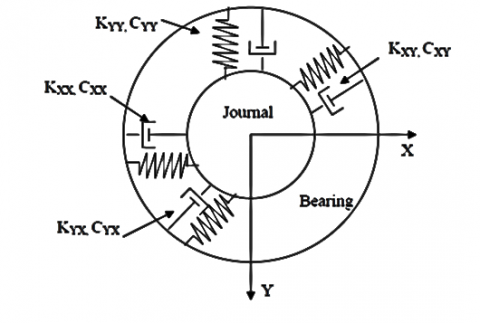Figure 2. Linearized-bearing coefficients

Table 2. Coded levels of the variable used in DOE

 factors Level 1 (high) Level 2 (low) Cyy 7.65E+06 3.64E+06 Cyz 4.66E+05 2.57E+06 Czy 4.66E+05 2.57E+06 Czz 4.43E+06 3.51E+06

## 3.pngFigure 3. Model-using Matlab

3.1 The Plackett Burman Design (PBD) method

Plackett and Burman . They explain how to build plans making it possible to study a great number of factors in few tests. The more model factors there are, the better the estimate of such errors, so it is uncommon for experimenters to use a larger PB design than is strictly necessary, thus getting higher quality information on the significance of each “real” factor. PB designs utilize two levels for each factor, the higher level being denoted “+” and the lower “_” as usual. A further feature of the PB method is that the + and _ signs for the individual trial experiments are assigned in a cyclical manner. Moreover, these factors may affect the system response interactively, i.e. the effect of one factor may depend on the levels of others. Any interactions must also be distinguished from random measurement errors. Therefore, it is more common to use partial factorial designs in which some information, especially about interactions, may be sacrificed in the interests of a manageable number of experiments. the responsible diameters, which produce great effects on frequency as well the responses, which increase or decrease the main effects. The mathematical model is then a model without interaction:

$\mathrm{y}=\mathrm{a}_{0}+\sum \mathrm{a}_{\mathrm{i}} \mathrm{x}_{\mathrm{i}}$     (7)

The statistical analysis of the model is the main step of the analysis of the results. In some cases, it based on mathematical analysis data as well as for the application of the Daniel or Lenth method. Its purpose is the statistical analysis of the factors that significantly affect the response observed. It is possible to break down the variation of a response from contributions made by each of the factors in a model from the following relationship:

$C T R j=\frac{\beta_{j}^{2}}{\sum_{j=1}^{k} \beta_{j}^{2}}$   (8)

With: CTRj the contribution of the factor j to the variation of the response. βj the coefficient of the model associated with factor j, k the number of factors in the study.

## 4.png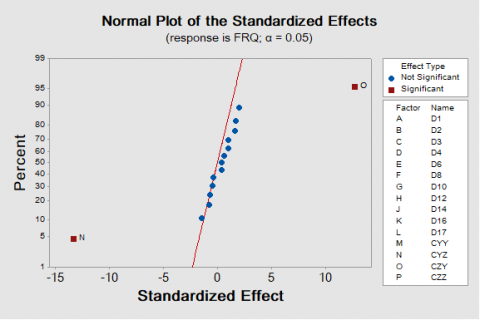Figure 4. Diagram of the standardized effect

At the initial stage, the results of the analysis for the factorial design on two levels and fifteen factors according to the design of Plakett-Burman in DOE from where the parameters used are the diameters and the damping coefficients of the hydrodynamic bearings. A response that is the frequency of excitation is obtained for each one with the support of MATLAB software. The DOE is carried out to obtain the effect of each damping coefficient on the frequency and the stability of the system and to determine which effects are the most significant coefficients evaluated by using α = 0,05 level of significance. In the graphics Figure 4, that Czy has a significant positive effect on the frequency but Cyz for a negative effect on the frequency that according to the position compared to the reference axis.

The contributions of the factors are then ascending ordered and presented in Figure 5.

## 5.png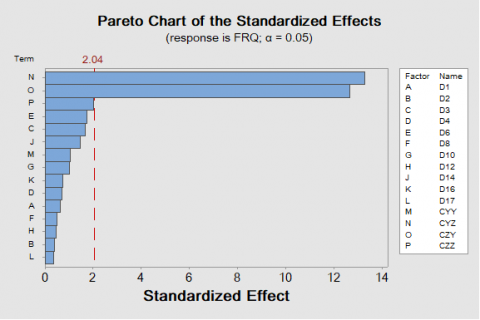Figure 5. Diagram of effect “Pareto”

Table 3. Effects and coefficients of frequencies

 Term Effect Coef SE Coef T-Value P-Value Constant 86.6542 0.0124 6981.84 0.000 D1 0.0158 0.0079 0.0124 0.64 0.528 D2 0.0092 0.0046 0.0124 0.37 0.714 D3 0.0408 0.0204 0.0124 1.64 0.110 D4 -0.0175 -0.0087 0.0124 -0.70 0.486 D6 0.0433 0.0217 0.0124 1.75 0.090 D8 -0.0117 -0.0058 0.0124 -0.47 0.642 D10 0.0250 0.0125 0.0124 1.01 0.321 D12 0.0108 0.0054 0.0124 0.44 0.665 D14 -0.0358 -0.0179 0.0124 -1.44 0.159 D16 -0.0183 -0.0092 0.0124 -0.74 0.466 D17 -0.0083 -0.0042 0.0124 -0.34 0.739 Cyy 0.0258 0.0129 0.0124 1.04 0.306 Cyz -0.3300 -0.1650 0.0124 -13.29 0.000 Czy 0.3150 0.1575 0.0124 12.69 0.000 Czz 0.0500 0.0250 0.0124 2.01 0.052

According to the experimental design of DOE and to determine what effects are significant of the coefficients evaluated using α = 0.05. In the Table 3, we can see that Cyz, Czy all significant since their p-values are less than alpha of 0.05. The factor having the highest effect coefficient in absolute value Czy (0.3150) has a greater impact on the given response. The standard coefficient error (SECoef) is constant which the error cannot estimate and should optimized

4. Results and Discussion

The design of the parameter is to identify arrangements of the parameters of the design, which optimize the operation and reduce the sensitivity of the studies. The design of the parameter requires evaluating the effect of the damping coefficients defined by values so that the parameters of the design choose the optimum levels so that the system is functioned and stable. The optimization based on the circular shifts of the matrix obtained. Thus, will accomplished as follows by moving the columns of basic matrix design manually and maintaining the values corresponding of frequency for each line and by conserving the balance of matrix. The matrix presented in Table 4. Shows 48 useful tests allowing optimization by Plakett-Burman design.

Table 4. Results obtained in DOE

 1 D1 D2 D3 D4 D6 D8 D10 D12 D14 D16 D17 CYY CYZ CZY CZZ FRQ 1 0.0152 0.0254 0.0102 0.0456 0.061 0.0458 0.0304 0.0354 0.0304 0.071 0.0356 7.65E+06 4.66E+05 2.57E+06 3.51E+06 8.69E+01 2 0.0152 0.0254 0.0204 0.0456 0.061 0.0558 0.0304 0.0354 0.0304 0.071 0.0356 3.64E+06 4.66E+05 4.66E+05 4.43E+06 8.67E+01 3 0.0152 0.0154 0.0102 0.0356 0.071 0.0558 0.0304 0.0354 0.0204 0.071 0.0456 3.64E+06 4.66E+05 2.57E+06 3.51E+06 8.71E+01 4 0.0152 0.0254 0.0204 0.0456 0.061 0.0558 0.0304 0.0354 0.0304 0.071 0.0356 3.64E+06 4.66E+05 4.66E+05 4.43E+06 8.67E+01 5 0.0152 0.0254 0.0102 0.0356 0.061 0.0458 0.0204 0.0254 0.0204 0.061 0.0456 7.65E+06 2.57E+06 4.66E+05 4.43E+06 8.65E+01 6 0.0052 0.0254 0.0204 0.0456 0.071 0.0458 0.0204 0.0254 0.0204 0.071 0.0456 3.64E+06 4.66E+05 4.66E+05 3.51E+06 8.67E+01 7 0.0152 0.0154 0.0204 0.0356 0.071 0.0558 0.0204 0.0254 0.0204 0.071 0.0356 7.65E+06 4.66E+05 4.66E+05 4.43E+06 8.67E+01 8 0.0152 0.0254 0.0204 0.0356 0.061 0.0458 0.0204 0.0354 0.0204 0.061 0.0356 3.64E+06 2.57E+06 4.66E+05 3.51E+06 8.62E+01 9 0.0152 0.0154 0.0102 0.0356 0.071 0.0558 0.0304 0.0354 0.0204 0.071 0.0456 3.64E+06 4.66E+05 2.57E+06 3.51E+06 8.71E+01 10 0.0152 0.0254 0.0204 0.0456 0.061 0.0558 0.0204 0.0254 0.0304 0.071 0.0356 7.65E+06 4.66E+05 2.57E+06 4.43E+06 8.68E+01 11 0.0052 0.0254 0.0204 0.0456 0.071 0.0458 0.0304 0.0354 0.0304 0.071 0.0356 7.65E+06 4.66E+05 4.66E+05 4.43E+06 8.67E+01 12 0.0052 0.0154 0.0102 0.0356 0.061 0.0558 0.0304 0.0354 0.0304 0.061 0.0356 7.65E+06 4.66E+05 4.66E+05 3.51E+06 8.67E+01 13 0.0152 0.0254 0.0102 0.0456 0.061 0.0558 0.0204 0.0254 0.0204 0.061 0.0456 3.64E+06 2.57E+06 2.57E+06 3.51E+06 8.66E+01 14 0.0052 0.0254 0.0204 0.0356 0.061 0.0558 0.0304 0.0354 0.0204 0.071 0.0356 7.65E+06 2.57E+06 2.57E+06 3.51E+06 8.67E+01 15 0.0152 0.0154 0.0204 0.0356 0.071 0.0458 0.0304 0.0254 0.0304 0.071 0.0456 7.65E+06 2.57E+06 2.57E+06 3.51E+06 8.67E+01 16 0.0152 0.0254 0.0204 0.0456 0.061 0.0558 0.0304 0.0354 0.0304 0.071 0.0356 3.64E+06 4.66E+05 4.66E+05 4.43E+06 8.67E+01 17 0.0152 0.0254 0.0102 0.0456 0.061 0.0558 0.0204 0.0254 0.0204 0.061 0.0456 3.64E+06 2.57E+06 2.57E+06 3.51E+06 8.66E+01 18 0.0152 0.0154 0.0204 0.0356 0.071 0.0558 0.0204 0.0254 0.0204 0.071 0.0356 7.65E+06 4.66E+05 4.66E+05 4.43E+06 8.67E+01 19 0.0152 0.0154 0.0204 0.0456 0.071 0.0458 0.0304 0.0254 0.0204 0.061 0.0356 3.64E+06 4.66E+05 2.57E+06 4.43E+06 8.70E+01 20 0.0052 0.0254 0.0204 0.0356 0.061 0.0558 0.0304 0.0354 0.0204 0.071 0.0356 7.65E+06 2.57E+06 2.57E+06 3.51E+06 8.67E+01 21 0.0052 0.0154 0.0102 0.0456 0.061 0.0558 0.0304 0.0354 0.0304 0.061 0.0356 7.65E+06 2.57E+06 2.57E+06 4.43E+06 8.67E+01 22 0.0152 0.0154 0.0102 0.0456 0.071 0.0558 0.0204 0.0354 0.0304 0.061 0.0456 3.64E+06 2.57E+06 2.57E+06 4.43E+06 8.66E+01 23 0.0052 0.0154 0.0102 0.0356 0.071 0.0558 0.0204 0.0254 0.0204 0.071 0.0456 3.64E+06 4.66E+05 2.57E+06 4.43E+06 8.70E+01 24 0.0052 0.0254 0.0204 0.0456 0.071 0.0458 0.0204 0.0254 0.0204 0.071 0.0456 3.64E+06 4.66E+05 4.66E+05 3.51E+06 8.67E+01 25 0.0152 0.0254 0.0204 0.0356 0.061 0.0458 0.0204 0.0354 0.0204 0.061 0.0356 3.64E+06 2.57E+06 4.66E+05 3.51E+06 8.62E+01 26 0.0052 0.0154 0.0204 0.0356 0.071 0.0558 0.0304 0.0354 0.0304 0.061 0.0356 7.65E+06 4.66E+05 2.57E+06 4.43E+06 8.70E+01 27 0.0052 0.0254 0.0102 0.0456 0.071 0.0458 0.0204 0.0354 0.0304 0.061 0.0456 3.64E+06 2.57E+06 4.66E+05 3.51E+06 8.62E+01 28 0.0052 0.0154 0.0204 0.0456 0.061 0.0558 0.0304 0.0254 0.0204 0.071 0.0356 7.65E+06 2.57E+06 4.66E+05 4.43E+06 8.65E+01 29 0.0052 0.0154 0.0102 0.0456 0.061 0.0558 0.0304 0.0354 0.0304 0.061 0.0356 7.65E+06 2.57E+06 2.57E+06 4.43E+06 8.67E+01 30 0.0052 0.0254 0.0204 0.0356 0.061 0.0558 0.0304 0.0354 0.0204 0.071 0.0356 7.65E+06 2.57E+06 2.57E+06 3.51E+06 8.67E+01 31 0.0052 0.0154 0.0204 0.0356 0.061 0.0458 0.0304 0.0254 0.0204 0.061 0.0356 3.64E+06 2.57E+06 2.57E+06 4.43E+06 8.66E+01 32 0.0052 0.0154 0.0102 0.0356 0.071 0.0558 0.0204 0.0254 0.0204 0.071 0.0456 3.64E+06 4.66E+05 2.57E+06 4.43E+06 8.70E+01 33 0.0152 0.0154 0.0102 0.0356 0.071 0.0458 0.0204 0.0254 0.0204 0.061 0.0456 7.65E+06 2.57E+06 4.66E+05 4.43E+06 8.65E+01 34 0.0052 0.0254 0.0102 0.0456 0.071 0.0458 0.0204 0.0354 0.0304 0.061 0.0456 3.64E+06 2.57E+06 4.66E+05 3.51E+06 8.62E+01 35 0.0152 0.0154 0.0102 0.0356 0.071 0.0458 0.0204 0.0254 0.0204 0.061 0.0456 7.65E+06 2.57E+06 4.66E+05 4.43E+06 8.65E+01 36 0.0052 0.0254 0.0204 0.0456 0.071 0.0458 0.0304 0.0354 0.0304 0.071 0.0356 7.65E+06 4.66E+05 4.66E+05 4.43E+06 8.67E+01 37 0.0152 0.0154 0.0204 0.0356 0.071 0.0458 0.0304 0.0254 0.0304 0.061 0.0456 7.65E+06 2.57E+06 4.66E+05 3.51E+06 8.64E+01 38 0.0152 0.0154 0.0204 0.0356 0.071 0.0458 0.0304 0.0254 0.0304 0.061 0.0456 7.65E+06 2.57E+06 4.66E+05 3.51E+06 8.64E+01 39 0.0152 0.0254 0.0102 0.0456 0.061 0.0458 0.0304 0.0354 0.0304 0.071 0.0356 7.65E+06 4.66E+05 2.57E+06 3.51E+06 8.69E+01 40 0.0152 0.0254 0.0102 0.0456 0.061 0.0458 0.0304 0.0354 0.0304 0.071 0.0356 7.65E+06 4.66E+05 2.57E+06 3.51E+06 8.69E+01 41 0.0052 0.0154 0.0204 0.0456 0.071 0.0458 0.0204 0.0254 0.0204 0.061 0.0456 3.64E+06 2.57E+06 2.57E+06 3.51E+06 8.66E+01 42 0.0052 0.0254 0.0102 0.0356 0.061 0.0558 0.0204 0.0254 0.0204 0.071 0.0456 3.64E+06 4.66E+05 2.57E+06 4.43E+06 8.70E+01 43 0.0152 0.0154 0.0102 0.0456 0.071 0.0558 0.0204 0.0354 0.0304 0.061 0.0456 3.64E+06 2.57E+06 2.57E+06 4.43E+06 8.66E+01 44 0.0052 0.0154 0.0102 0.0356 0.061 0.0558 0.0304 0.0354 0.0304 0.061 0.0356 7.65E+06 4.66E+05 4.66E+05 3.51E+06 8.67E+01 45 0.0052 0.0154 0.0102 0.0356 0.061 0.0458 0.0204 0.0254 0.0304 0.061 0.0456 3.64E+06 2.57E+06 4.66E+05 4.43E+06 8.63E+01 46 0.0052 0.0254 0.0102 0.0456 0.071 0.0458 0.0204 0.0254 0.0304 0.061 0.0456 7.65E+06 4.66E+05 2.57E+06 3.51E+06 8.69E+01 47 0.0052 0.0154 0.0102 0.0356 0.061 0.0458 0.0204 0.0254 0.0304 0.061 0.0456 3.64E+06 2.57E+06 4.66E+05 4.43E+06 8.63E+01 48 0.0052 0.0254 0.0204 0.0456 0.071 0.0458 0.0204 0.0254 0.0204 0.071 0.0456 3.64E+06 4.66E+05 4.66E+05 3.51E+06 8.67E+01

4.1 Result of optimization (PBD)

4.1.1 Effects and coefficients of frequencies

Table 5 below, the optimization shows that for each main effect term and each interaction term, there is a coefficient and main effect twice the Coefficient. To determine the relative strength of the factors, will be examine the effects and the coefficients, the value and the signs are both important in the determination. can be observed that Czy has the greatest effect on the response and the value of the Standard coefficient error (0.000822) clarifies a higher response of more the estimate is accurate and Cyz has the smallest effect and the value of the standard coefficient error (0.00134) results in a higher Response, we follow the same approach in analysis the factors. The standard coefficient error (0.0007) clarifies the model is Adjusted with the data and who concludes from the results presented.

All terms are significant since all values of p for these terms are less than 0.05. The sign of the coefficient indicates the meaning of the relation between the term and the response. The importance of the coefficient corresponds to half of that of the effect. The effect represents the expected change in the mean response as the factor moves from its level lower than its upper level. The effect value usually helps to assess whether the effect of a term on the response variable is meaningful in practice.

Table 5. Effects and coefficients of frequencies

 Term Effect Coef SE Coef T-Value p-Value Const 86.6542 0.0007 129245.90 000 D1 0.015833 0.007917 0.000670 11.81 0. 000 D2 -0.04922 -0.02461 0.00148 -16.66 0.000 D3 0.05194 0.02597 0.00157 16.53 0.000 D4 0.030585 0.015293 0.000992 15.41 0.000 D6 -0.05552 -0.02776 0.00176 -15.81 0.000 D8 0.02588 0.01294 0.00100 12.93 0.000 D10 0.13607 0.06803 0.00125 54.21 0.000 D12 0.06669 0.03334 0.00179 18.65 0.000 D14 -0.14690 -0.07345 0.00111 -65.90 0.000 D16 0.04160 0.02080 0.00110 18.89 0.000 D17 0.24370 0.12185 0.00236 51.73 0.000 CYY 0.09736 0.04868 0.00124 39.21 0.000 CYZ -0.39518 -0.19759 0.00134 -146.99 0.000 CZY 0.278799 0.139400 0.000822 169.56 0.000 CZZ 0.092739 0.046369 0.000917 50.56 0.000

4.1.2 Analysis of variance

In Table 6 the analysis of Variance shows that all the terms are significant since all the values of p for these terms are lower than α = 0.05. The larger the F value1722.80, the greater the effect on the performance characteristic . It can see that the Cyz, Czy parameters have significant significance.

Table 6. Variance analysis of frequency

 Source DF Adj SS Adj MS F-Value P-Value Model 15 2.84964 0.189976 1722.80 0.000 Linear 15 2.84964 0.189976 1722.80 0.000 D1 1 0.00301 0.003008 27.28 0.000 D2 1 0.00315 0.003152 28.58 0.000 D3 1 0.00439 0.004392 39.83 0.000 D4 1 0.00393 0.003934 35.68 0.000 D6 1 0.00323 0.003232 29.31 0.000 D8 1 0.00427 0.004273 38.75 0.000 D10 1 0.06272 0.062724 568.81 0.000 D12 1 0.00533 0.005329 48.33 0.000 D14 1 0.08770 0.087703 795.34 0.000 D16 1 0.00936 0.009358 84.87 0.000 D17 1 0.05413 0.054131 490.89 0.000 CYY 1 0.02944 0.029436 266.94 0.000 CYZ 1 0.48275 0.482752 4377.84 0.000 CZY 1 0.61356 0.613565 5564.12 0.000 CZZ 1 0.04730 0.047305 428.98 0.000 Error 23 0.00353 0.000110 Lack-of-Fit 11 0.00353 0.000321 Pure Error 21 0.00000 0.000000 Total 47 2.8531

Table 7 presents that `S’ has a small value, the model describes the response, and More the values R 2 , R- adjusted square and the predicted R-square are that more than 90%, the model is adjusted with the data and who concludes are a desirable characteristic from the results presented.

Figure 6 shows the absolute values of the normalized effects but not the ones that increase or decrease the response and allow to identify important effects and to compare the relative importance of the various effects. The main effects have a significant impact, since the bars that represent these terms on the graph has extended beyond the red vertical reference line at the default level of 0.05. More clearly, Czy and Cyz have high main effects. In addition, it can see that the maximum effect is that of Czy, because it is the one with the largest bar.

Table 7. Summary of adjustment

 S R-sq R-sq(adj) R-sq(pred) PRESS 0.0046451 100% 99.96% 99.94% 0.0016297

## 6.png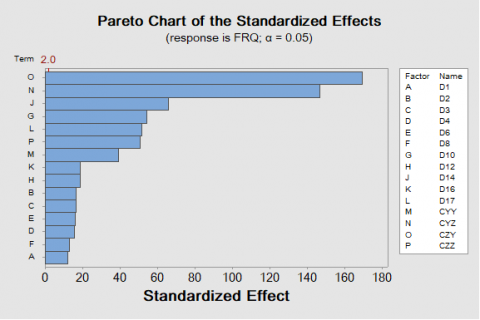Figure 6. Diagram of the absolute value of the effects “Pareto”

## 7.png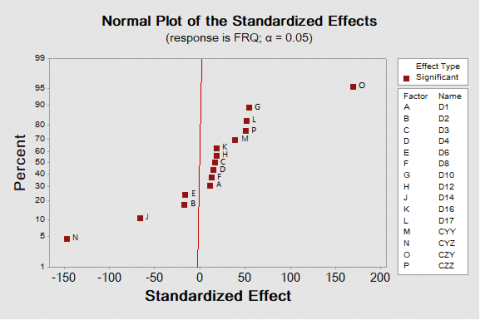Figure 7. Normal diagram of the effects

The effects diagram shows in Figure 7. To study the amplitude, direction and importance of the effects on the same graph marks a reference line where statistically negative effects on the left side of the graph and the effects statistically positive on the right side, more the effects are distant from the zero on axis X, are statistically significant and their amplitude is high. The factor Czy (O) has a positive standardized effect. When Czy (O) vary from the level lower at the higher level of the factor, the response increases. Of it the Factor Cyz (N) has a negative effect when this factor increases, the response decreases. In addition, the diameter D10 (G) is statistically significant. The half-diagram of effect in Figure 8 shows the absolute values of the effects standardized, according to classifying the higher with feeble. The effects most distant from zero are statistically more significant and their amplitude is high.

## 8.png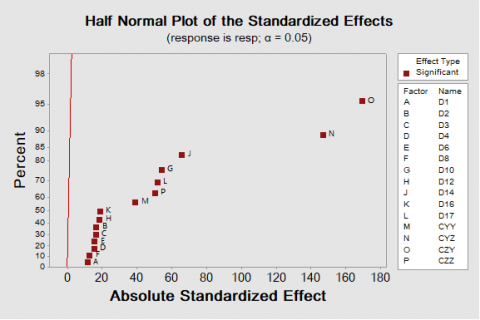Figure 8. Half-Normal diagram of the effects

Figure 9 below displays the principal lines of effect of various parameters and indicate the influence of each factor on the excitation frequency observed. The damping coefficients Czy, Cyy, Czz, the diameters, D17, D12, and D10 have effects significant on the frequency. The results of the graph indicated that the damping coefficient Czy has a principal effect on the frequency as compared with Cyy, Czz and Cyz and the diameter D17 has a main effect on the frequency as compared to D12, D10 and D14. The whole of the factor (diameters D1, D2, D4, D6, D8, and D16) do not have a significant influence on the frequency of the excitation. In addition, the main effects cannot be interpreted without taking into account the effects of interactions.

The Minitab interactions in which the effect of a factor depends on the level on the other factor where the levels factors indicated on the horizontal axis and the levels of the other factor specified by lines and symbols of different colors. The parallel lines indicate the absence of interactions and as the difference in slope is getting larger between the lines, the degree of interaction is getting higher. However, the diagram of the interactions does not indicate if the interaction is statistically significant. Figure 10 and 11 indicates the interactions between the factors studied who explicates that the interaction of Cyz and Cyy shows that as Cyz values vary from 2,5740E+06 to4,6634E+06 the frequency decreases, while Cyy values vary from 3,6434E+06 to 7,6527E+06 the frequency decreases. The Czy and Cyy interaction shows the Czy values differ from 2,5740E+06 to 4,6634E+05 the frequency increases and that the Cyy value differ from3,6434E+06 to7,6527E+06the frequency decreases. In addition, The Czz and Cyy interaction show the Czz values increase the frequency increase and the Cyy value increases, the frequency increases. The same approach is being done in analysis and the results of interactions (Cyy, Cyz), (Cyy, Czy). (D17, Cyy), (D16, Cyy), (D16, D17), (D14, Cyy), (D14, D17), (D12, D10), (D10, Cyy), can increase or decrease the frequency.

## 9.png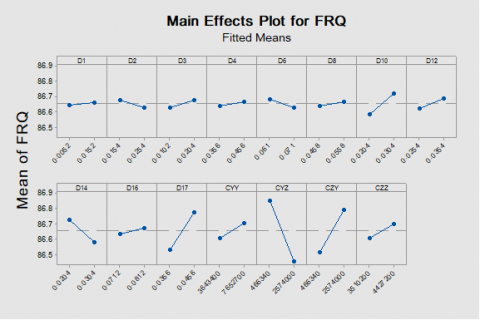Figure 9. Graph of the principal effects

## 10.png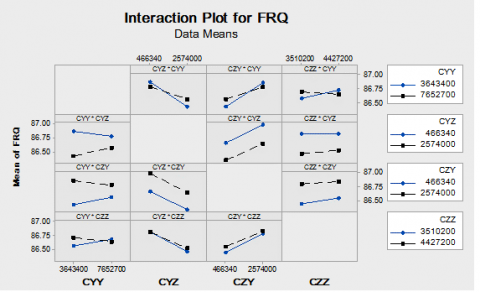Figure 10. Diagram of interaction for the frequency

## 11.png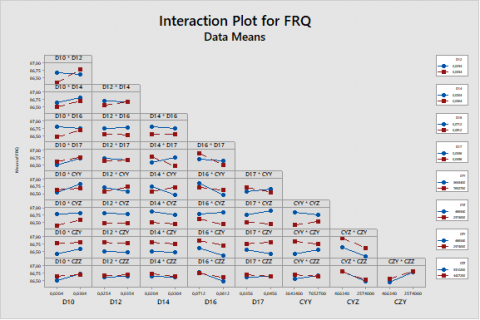Figure 11. Interaction plot total for frequency

## 12.png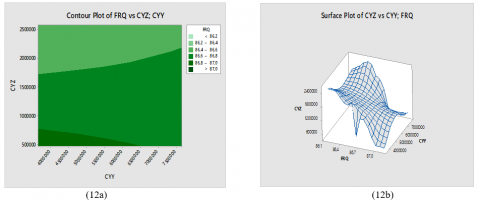Figure 12. Contour plot (12a) and surface plot (12b) of Cyz, and Cyy of frequency

## 13.png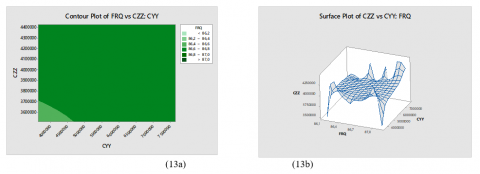Figure 13. Contour plot (13a) and surface plot (13b) of CZZ and Cyy of frequency

## 14.png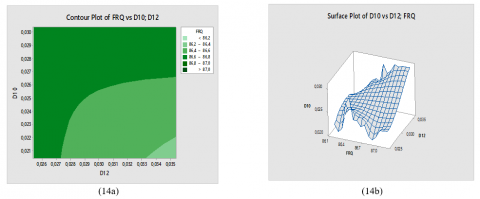Figure 14. Contour plot (14a) and surface plot (14b) of D10 and D12 of frequency

## 15.png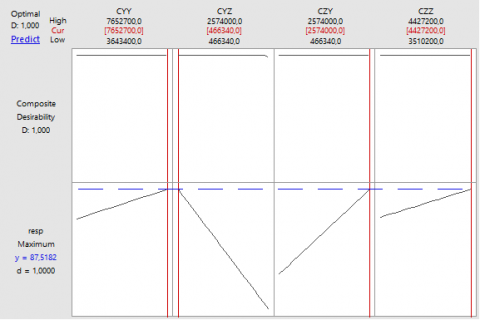Figure 15. Optimum solution for hydrodynamic bearings

In Figure 12(a) to 14(a) a contour plot, the values for two variables are represented on the x- and y-axes, while the values for a third variable are represented by shaded regions and the response surface in the three-dimensional are shown in Figures 12(b) to 14(b). These surfaces plot display a continuous grid representing response values based on the mesh. Minitab estimates the values of response variable at the mesh intersections using the model-fitting procedure where the mesh can edit to see higher or lower resolution to get the fitted surface. In the graphs, can be observe in Figure 12 that the frequency are greater than 87when Cyy values decrease and the frequency values are less than 86.2 when the Cyz values increase, in Figure 13 the frequency values are greater than 87 when Cyy values increase and the frequency values are less than 86.2 when the Czz values decrease. The same analysis of the interaction (D10, D12), (D16, Cyy), (D16, D17), (D14, Cyy), (D14, D17), (D17, Cyy), (D10, Cyy), which can increase or decrease the frequency.

The optimization diagram Figure 15 describes the optimal design carried and shows the effect of each factor on the responses of the four objective coefficients. The diagram indicates that the damping coefficients are wide with a positive effect of slope, and Cyz has a negative linear effect of slope but the response vary linearly, and the Composite desirability was one 1 appreciate that the final design was very favorable for all the responses.

However, the individual desirability indicate that arrangements are more effective to the maximum (Cyz, Czy, Cyy, Czz). Minitab calculates the predicted response (87.5182) when all the factors are with their higher values. Therefore, to obtain this desirability we would place the levels of factor at the values shown under the solution in red on the diagram.

5. Conclusions

In this research, a methodology of DOE was selected to optimize the principal parameters affecting the dynamic characteristics of the hydrodynamic bearing particularly the damping coefficients on the stability of the system and to knowing the parameters responsible for creating a great effect, which reduce or increase the frequency. This study shows a Fractional factorial designs by the method of Plackett-Burman that is employ to optimize the execution of the parameter of the system. The results show that CZY has the greatest significant positive effect on the frequency and Cyz has a negative impact on the frequency, as presented by the variance analysis in the graphs and the results of the analysis. In addition, the diameter D10 andD17 have an influence significant on the frequency. The major effect on the frequency of optimal excitation of the parameters was determined about the diagram of optimization and the surface of response the predicted response (87.5182) when all the factors are with their higher values. These results show that the insert the statistic methods in calculate instabilities of the revolving machines allow profit of time and quality in term to control the system.

Further study could consider more factors to see how they influence, such as dimensional effects, tree material ... etc. for balancing and stability of rotating machines. Future work can be done on the application of the DOE method for the optimization of other processes in industry

Nomenclature
 wy wx Hydrodynamic components of the bearing $\dot{y}$, z. Velocity components. a0 ai gravitational acceleration, m.s-2 Linear coefficient DF Degrees of freedom from each source SS Sum of squares. MS Mean squares. F Calculate by dividing the factor MS by error. P Use to determine whether a factor is signif. Secoff Standard error of the coefficient. S Estimated standard deviation of the error. Seq SS Sequential sum of squares. Adj SS Adjusted sum of squares. F The degrees of freedom for the test Cij Damping coefficients ij(xy).
References

 Newkirk, B.L., Lewis, J.F. (1925). Shaft whipping due to oil action in journal bearings. General Electric Review, XXVIII(8): 559-568.

 Cha, M., Evgeny, K., Glavatskih, S. (2013). A comparative linear and nonlinear dynamic analysis of compliant cylindrical journal bearings. Journal of Mechanism and Machine Theory, 64: 80-92. https://doi.org/10.1016/j.mechmachtheory.2013.01.008

 Goupy, J. (2001). Introduction aux Plans d'expériences. Dunod, Paris.

 Sinou, J.J., Viana, C., Thouverez, F. (2005). Experimental and numerical investigations of a flexible rotor on flexible bearing supports. International Journal of Rotating Machinery, 2005(3): 179-189. https://doi.org/10.1155/IJRM.2005.179

 Khonsari, M.M., Chang, Y.J. (1993). Stability boundary of non-linear orbits within clearance circle of journal bearings. ASME Journal of Vibration and Acoustics, 115(3): 303-307. https://doi.org/10.1115/1.2930349

 Lu, Y.J., Zhang, Y.F., Dai, R., Liu, H., Yu, L., Hei, D., Wang, Y. (2008). Non-linear analysis of a flexible rotor system with multi-span bearing supports. Proceedings of the Institution of Mechanical Engineers, Part J: Journal of Engineering Tribology, 222(2): 87-95. https://doi.org/10.1243/13506501JET308

 Vazquez, J.A., Barrett, L.E., Flack, R.D. (2001). A flexible rotor on flexible bearing supports: Stability and unbalance response. Journal of Vibration and Acoustics, 123(20): 137-144. https://doi.org/10.1115/1.1355244

 Edwards, S., Lees, A.W., Friswell, M.I. (2001). Experimental identification of excitation and support parameters of a flexible rotor bearings foundation system from a single run down. Journal of Sound and Vibration, 232(5): 963-992. https://doi.org/10.1006/jsvi.1999.2779

 Lees, A.W., Friswell, M.I. (1997). The evaluation of rotor imbalance in flexibly mounted machines. Journal of Sound and Vibration, 208(5): 671-683. https://doi.org/10.1006/jsvi.1997.1260

 Kaidi, I., Kabouche, A. (2018). Analyse du défaut de balourd dans un palier hydrodynamique. Synthèse: Revue des Sciences et de la Technologie, 36: 87-102.

 Chouksey, M., Dutt, J.K., Modak, S.V. (2011). Modal analysis of rotor-shaft system under the influence of rotor-shaft material damping and fluid film forces. Mechanism and Machine Theory, 48(1): 81-93. https://doi.org/10.1016/j.mechmachtheory.2011.09.001

 Mostefa, B., Kaddour, R., Mimoun, Y., Abdallah, N. (2018). Plackett-burman desing to study the influence of the stiffness of hydrodynamic bearings on the dynamic behaviour of turbo machinery. Mathematical Modelling of Engineering Problems, 5(4): 407-417. https://doi.org/10.18280/mmep.050418

 Fegade, R., Patel, V. (1521). Unbalanced response and design optimization of rotor by ANSYS and design of experiments. International Journal of Scientific & Engineering Research, 4(7).

 Li, K., Liu, J., Han, X., Jiang, C., Qin, H.J. (2016). Identification of oil-film coefficients for a rotor-journal bearing system Based on equivalent load reconstruction. Tribology International, 104: 285-293. https://doi.org/10.1016/j.triboint.2016.09.012

 Lund, J.W., Thomsen, K.K. (1978). A calculation method and data for the dynamic coefficients of oil-lubricated journal bearing. Topics in Fluid Film Bearing and Rotor Bearing System Design and Optimization, ASME New York, pp. 1-28.

 Nelson, H.D., Myers, R.H. (1976). The dynamics of rotor bearing system using finite elements. ASME Journal of Engineering for Industry, 98(2): 593-600.

 Myers, R.H., Montgomery, D.C. (1995). Response surface methodology. Process and Product Optimization Using Designed Experiments. John Wiley&Sons, Inc., NY, USA.

 Plakett, R.L., Burman, J.P. (1946). The design of optimum multifactorial experiments. Biometrika, 33(4): 305-325. https://doi.org/10.2307/2332195

 Venkata Sivareddy, D., Vamsi Krishna, P., Venu Gopal, A., Prithvi Raz, C.L. (2018). Parameter optimisation in vibration assisted turning of Ti6Al4V alloy using ANOVA and grey relational analysis. International Journal of Automotive and Mechanical Engineering, 15(3): 5400-5420. https://doi.org/10.15282/ijame.15.3.2018.1.0416